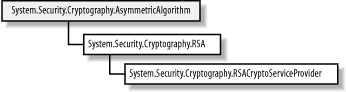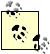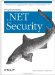# 15.2 Programming Asymmetrical Encryption

The .NET Framework takes the same approach to representing asymmetric algorithms as it does for symmetric algorithms and hashing algorithms; abstract classes extend the System.Security.Cryptography.AsymmetricAlgorithm class for each of the supported algorithms. Individual implementations of the algorithms extend the abstract class, supporting the possibility of more than one implementation of an algorithm. Figure 15-6 shows the class hierarchy that supports asymmetric encryption algorithms, which is very simple because the .NET Framework supports only the RSA algorithm.

##### Figure 15-6. The .NET Framework class hierarchy for asymmetric encryption algorithms#### 15.2.1 The AsymmetricAlgorithm class

Different asymmetric algorithms can take very different approaches to encrypting data, and the abstract AsymmetricAlgorithm class provides very little in the way of common functionality. Table 15-2 lists the pubic methods of the AsymmetricAlgorithm class.

##### Table 15-2. Members of the AsymmetricAlgorithm class

Member

Description

Properties

KeySize

Gets or sets the size in bits of the key modulus used by the algorithm

LegalKeySizes

Gets the key sizes supported by the algorithm

KeyExchangeAlgorithm

Gets the name of the key exchange algorithm

SignatureAlgorithm

Gets the name of the digital signature algorithm; see Chapter 16 for details of digital signatures

Methods

Create

Creates a new instance of the AsymmetricAlgorithm class by name

ToXmlString

Creates an XML string representation of the AsymmetricAlgorithm instance

FromXmlString

Reconstructs an instance of AsymmetricAlgorithm from an XML string

#### 15.2.2 The RSA class

The RSA class is the abstract representation of the RSA algorithm and extends AsymmetricAlgorithm. This class provides the common functionality shared by any implementation of the underlying algorithm and allows the algorithm to be employed without regard to the implementation details; however, as you will see in the following sections, the RSA class is not as useful as it should be. Table 15-3 summarizes the public members of the RSA class.

##### Table 15-3. Members of the RSA class

Method

Description

DecryptValue

Abstract decryption method consult the following sections for details of decrypting data with the RSA implementation class

EncryptValue

Abstract encryption method consult the following sections for details of encrypting data with the RSA implementation class

ExportParameters

Exports the public or private key using the RSAParameters structure

ImportParameters

Imports a key using the RSAParameters structure

The EncryptValue and DecryptValue methods are not as useful as they seem, as we will explain in the next section. The value of the RSA class is the addition of the ImportParameters and ExportParameters methods, which allow the public or private keys to be imported into or exported from an instance of the class. Table 15-4 details the members of the RSAParameters structure, which represents RSA keys as a related series of byte arrays.

##### Table 15-4. Members of the RSAParameters structure

Field

Description

Modulus

The key modulus value, n.

Exponent

The key exponent value, e.

D

The secret key value, d.

P

These values are used to increase the performance of the RSA algorithm.

Q

DP

DQ

InverseQ

You will be familiar with the purpose of the RSAParameters values Modulus, Exponent, and D from Section 15.1.1 in this chapter. The other fields represented by the structure can be used to increase the performance of the decryption function. The performance enhancement is not part of the RSA algorithm specification, but the RSA class only imports private keys using RSAParameters structures that contain all of the addition values. You do not need to understand how the performance enhancements work, but you should be aware that the .NET RSA classes always expect these additional values.

#### 15.2.3 The RSACryptoServiceProvider Class

This class is the only asymmetric encryption implementation class contained in the .NET Framework. Unfortunately, while this class extends the abstract RSA class, it does not implement the EncryptValue and DecryptValue methods, meaning that you must work with the implementation class directly to use the RSA algorithm. Table 15-5 details the new methods introduced by the RSACryptoServiceProvider class.

##### Table 15-5. Members of the RSACryptoServiceProvider class

Method

Description

Encrypt

Encrypts plaintext, using OAEP or PKCS #1 v1.5 padding.

Decrypt

Decrypts OAEP or PKCS #1 v1.5 padded ciphertext.

SignData

These methods create and verify digital signatures, which we discuss in Chapter 16.

SignHash

VerifyData

VerifyHash

#### 15.2.4 Instantiating the Algorithm

The RSACryptoServiceProvider class does not implement all of the methods defined by the abstract RSA class. There is no purpose in using the Create method specified in the AsymmetricAlgorithm class; the abstraction advantages offered with symmetric and hashing algorithms do not apply to RSA encryption, because you must always create instances of the implementation class to be able to use the Encrypt and Decrypt methods:

`# C# RSACryptoServiceProvider x_alg = new RSACryptoServiceProvider(  ); # Visual Basic .NET Dim x_al2g As RSACryptoServiceProvider = New RSACryptoServiceProvider(  )`

#### 15.2.5 Using RSA Keys

Even though you cannot use the abstract classes to encrypt and decrypt data, the methods that they define do allow you to configure the keys used by instances of the RSACryptoServiceProvider class.

The AsymmetricAlgorithm class provides the LegalKeySizes property, which returns an array of the KeySizes structure, specifying all of the key lengths that the algorithm supports. This is the same model used by the SymmetricAlgorithm class (see Section 14.3.3.1 of Chapter 14 for full details). The .NET RSA implementation supports key lengths from 384 to 16384 bits, in 8-bit steps, and the size of key you wish to use is set using the KeySize property.

The RSA implementation will create a new key pair automatically if you have not imported a key value before you encrypt or decrypt data. You can export the details of this key using the ExportParameters method, which returns an instance of the RSAParameters structure. This method accepts a Boolean argument. If the argument is true, then the private key is exported; otherwise, only the details of the public key are included:

`# C# RSACryptoServiceProvider x_alg = new RSACryptoServiceProvider(  );          // export only the public key  RSAParameters x_public_params = x_alg.ExportParameters(false); // export the private key  RSAParameters x_private_params = x_alg.ExportParameters(true); # Visual Basic .NET Dim x_alg As RSACryptoServiceProvider = New RSACryptoServiceProvider(  ) ' export only the public key  Dim x_public_params As RSAParameters = x_alg.ExportParameters(False) ' export the private key  Dim x_private_params As RSAParameters = x_alg.ExportParameters(True)`

The most common use for the ExportParameters method is to export details of a key from one instance of an algorithm and then import them to another using the ImportParameters method:

`# C#  // create an instance of the RSA algorithm RSACryptoServiceProvider x_alg = new RSACryptoServiceProvider(  ); // export only the public key  RSAParameters x_public_params = x_alg.ExportParameters(false); // create a second instance of the algorithm RSACryptoServiceProvider x_second_algorithm = new RSACryptoServiceProvider(  ); // import the public key x_second_algorithm.ImportParameters(x_public_params); # Visual Basic .NET ' create an instance of the RSA algorithm Dim x_alg As RSACryptoServiceProvider = New RSACryptoServiceProvider(  ) ' export only the public key  Dim x_public_params As RSAParameters = x_alg.ExportParameters(False) ' create a second instance of the algorithm Dim x_second_algorithm As RSACryptoServiceProvider = New RSACryptoServiceProvider(  ) ' import the public key x_second_algorithm.ImportParameters(x_public_params)`

The RSAParameters structure is also useful if you need to export a key into a custom format. The following statements demonstrate how to export the public part of a key pair and write it out as a pair of hexadecimal strings:

`# C# // create an instance of the RSA algorithm RSACryptoServiceProvider x_alg = new RSACryptoServiceProvider(  ); // export only the public key  RSAParameters x_public_params = x_alg.ExportParameters(false); Console.Write("n: "); foreach (byte x_byte in x_public_params.Modulus) {     Console.Write("{0:X2} ", x_byte); } Console.WriteLine(  ); Console.Write("e: "); foreach (byte x_byte in x_public_params.Exponent) {     Console.Write("{0:X2} ", x_byte); } # Visual Basic .NET ' create an instance of the RSA algorithm Dim x_alg As RSACryptoServiceProvider = New RSACryptoServiceProvider(  ) ' export only the public key  Dim x_public_params As RSAParameters = x_alg.ExportParameters(False) Console.Write("n: ") Dim x_byte As Byte For Each x_byte In x_public_params.Modulus     Console.Write("{0:X2} ", x_byte) Next x_byte Console.WriteLine(  ) Console.Write("e: ") For Each x_byte In x_public_params.Exponent     Console.Write("{0:X2} ", x_byte) Next x_byte`

The ToXmlString method exports the key information as an XML string, as in the following example:

`<RSAKeyValue>    <Modulus>xCDce...</Modulus>    <Exponent>AQAB</Exponent>    <P>6heANKwqXC4d...</P>    <Q>1nvPzfGay26Q...</Q>    <DP>Wxyyhc3M3vw...</DP>    <DQ>i0evdC8WtFB...</DQ>    <InverseQ>r01lw... </InverseQ>    <D>mHKmjx5hjhZn...</D> </RSAKeyValue>`

The XML string represents each of the values from the RSAParameters structure as a Base64-encoded string; we have shortened the strings shown, which are very long even for a 384-bit key. The ToXmlString method accepts a Boolean argument that includes the private key when set to true and exports only the public key when false. You can import XML strings exported using this method into instances of RSACryptoServiceProvider using the FromXmlString method.

#### 15.2.6 Encrypting and Decrypting Data

The RSACryptoServiceProvider class defines the Encrypt and Decrypt methods, each of which processes a byte array, as demonstrated by the following statements:

`# C# using System; using System.Security.Cryptography; using System.Text; class RSAExample {             static void Main(  ) {         // define the plaintext to encrypt         byte[] x_plaintext              = Encoding.Default.GetBytes("Programming .NET Security");         // create an instance of the RSA algorithm         RSACryptoServiceProvider x_alg = new RSACryptoServiceProvider(  );         // encrypt the plaintext using OAEP padding         byte[] x_ciphertext = x_alg.Encrypt(x_plaintext, true);         // decrypt the plaintext         byte[] x_new_plaintext = x_alg.Decrypt(x_ciphertext, true);         // reconstruct a string from the new plaintext and print it out         string x_output = Encoding.Default.GetString(x_new_plaintext);         Console.WriteLine(x_output);     } } # Visual Basic .NET Imports System Imports System.Security.Cryptography Imports System.Text Class RSAExample     Shared Sub Main(  )         ' define the plaintext to encrypt         Dim x_plaintext As Byte(  ) _         = Encoding.Default.GetBytes("Programming .NET Security")         ' create an instance of the RSA algorithm         Dim x_alg As RSACryptoServiceProvider = New RSACryptoServiceProvider(  )         ' encrypt the plaintext using OAEP padding         Dim x_ciphertext(  ) As Byte = x_alg.Encrypt(x_plaintext, True)         ' decrypt the plaintext         Dim x_New_plaintext(  ) As Byte = x_alg.Decrypt(x_ciphertext, True)         ' reconstruct a string from the new plaintext and print it out         Dim x_output As String = Encoding.Default.GetString(x_New_plaintext)         Console.WriteLine(x_output)     End Sub End Class`

The Boolean argument to the Encrypt and Decrypt methods specifies whether to use OAEP padding; if this value is set to false, then PKCS #1 v1.5 padding will be used.The OAEP padding mode is available only on computers running Windows XP or earlier versions upgraded with the "high encryption" pack.

The RSA implementation does not support processing data using the CryptoStream class that we described in Chapter 14. The relatively slow performance of all asymmetric algorithms means that they are rarely used to encrypt large amounts of data and are most frequently employed for "key exchange," which we discuss in Chapter 17.Programming .Net Security
ISBN: 0596004427
EAN: 2147483647
Year: 2005
Pages: 346

Similar book on Amazon